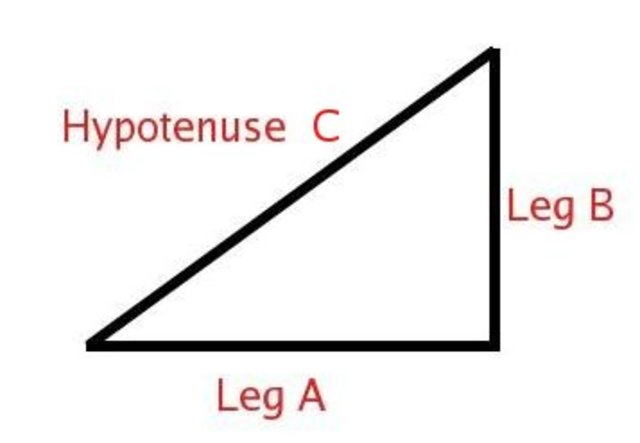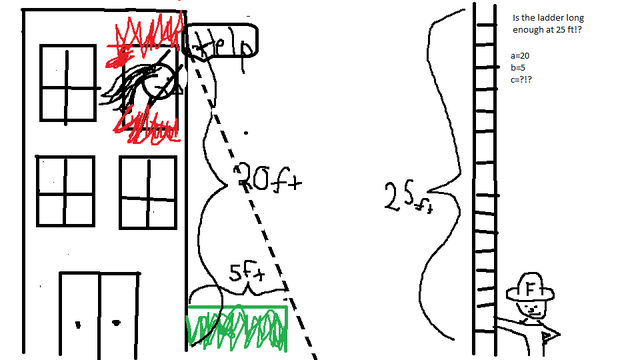• 570

# PythagorasPythagoras was born in 570BC and lived until 495 BC. He is credited with finding, and the proof of, the Pythagorean Theorom.
• 570

# The EquationThe Pythagorean Theorom definition: In any right-angled triangle, the area of the square whose side is the hypotenuse is equal to the sum of the areas of the squares whose sides are the two legs. From this definition we can form the equation, a^2+b^2=c^2 where c is the hypotenuse.
• 570

# Solving the equation step 1Find one leg of your right triangle, not the hypotenuse. This will be a.
• 570

# Solving the Equation Step 2

After you have found a, sqaure it. (Multiply it by itsself). This is your value for a.
• 570

# Solving the Equation Step 3Find the second leg on the right triangle, not the hypotenuse. This will be b.
• 570

# Solving the Equation Step 4

After you have found side b, square it (multiply it by itsself). This is your value for b.
• 570

# Solving the Equation Step 5Take your values for a and b, then add them together. This value is your c^2 value.
• 570

# Solving the Equation Step 6Take the square root of your c ^2value to find the value of c. You have found your hypotenuse!
• 570

# Example of a Solved Equation

a^2+b^2=c^2 a=5
b=8
c=? a= 5x5
b=8x8 25+64=c^2 89=c^2 √89 = 9.43 <-- This is the length of they hypotenuse.
• 570

# Real Life Example of Pythagorean TheoremImagine that you're a firefighter. There is a woman in a burning building on the 2nd floor. There is also a hedge that pertrudes about 5 feet from the base of the building. Your ladder is 25 feet long. Will your ladder reach the lady?